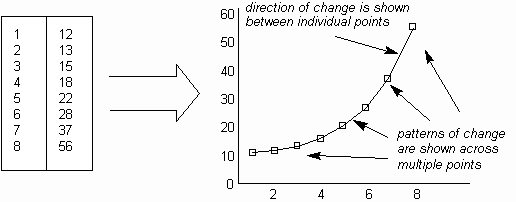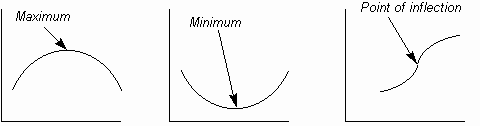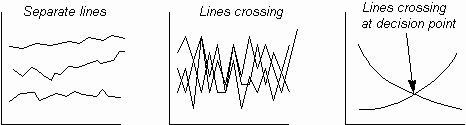The Psychology of Quality and More

# Line Graph: How to understand it

The Quality ToolbookLine Graph > How to understand it

## How to understand it

When taking measurements of a process, the result is often a simple list of numbers. Although this list may be interpreted to some degree by examination, the information contained in the numbers can often be made easier to understand by showing the numbers in a Line Graph.

The points on the graph are plotted from pairs of numbers in the list, with lines drawn between each pair, as in The illustration. Typically, one number in the pair is the measured item and is shown on the vertical axis, whilst the second number, shown on the horizontal axis, indicates either the time or sequence number of the measurement.

A Line Graph highlights the relative change between individual measurement points through the slope (or gradient) of the line drawn between them, as in The illustration. The change across a number of points can be seen through the overall shape of the graph.When the graph is drawn, patterns of change may then be identified and interpreted. Fig. 1. shows some typically significant patterns:

• A trend shows an overall movement in one direction, which may be masked by the ups and downs of individual points. For example, where daily sales figures go up and down, but general sales slowly increase over the longer term.
• A spike is a short term change, which may be caused by some unusual event, such as a weak batch of yeast causing a large number of rejections in a bakery.
• A step is a sudden and persistent change. For example, where a silicon doping process is improved, resulting in a sharply increased yield in integrated circuit chips.Fig. 1. Trends, spikes and steps

When a line forms a curve, then the change in slope of the curve may be significant. Decision points thus commonly occur at maxima, minima or points of inflection (where the rate of change in the slope reverses). These are shown in The illustration.Fig. 2. Curve change points

Multiple Line Graphs, can be useful for showing multiple sets of measurements, either to save graph space or to compare measurement sets, but they become unusable where lines get confused through crossing one another. Line crossing is useful where this highlights a decision points. These are illustrated in The illustration.Fig. 3. Multiple lines

Generally, when interpreting Line Graphs, if the expected shape of the graph is known, then it becomes easier to spot significant decision points along the plotted lines.

### You can buy books here

And the big
paperback book